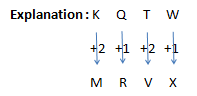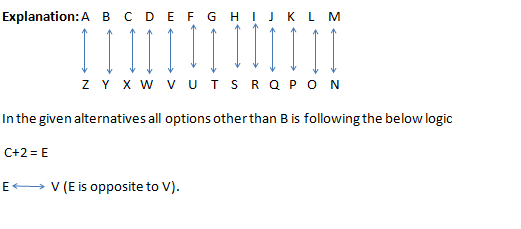# SSC CGL 2018 Practice Test Papers | Reasoning Ability (Day-3)

Dear Aspirants, Here we have given the Important SSC CGL Exam 2018 Practice Test Papers. Candidates those who are preparing for SSC CGL 2018 can practice these questions to get more confidence to Crack SSC CGL 2018 Examination.

[WpProQuiz 2650]

Click “Start Quiz” to attend these Questions and view Explanation

1. In the following question, select the related word from the given alternatives.

Apricot : Fruit : : Clove : ?

1. Seed
2. Fruit
3. Bud
4. Stem
1. In the following question, select the related letters from the given alternatives.

KQTW: MRVX:: NSGT : ?

1. PUVU
2. PTIU
3. OTHV
4. OPHS
1. In the following question, select the related number from the given alternatives.

257 : 626 : : 1297 : ?

1. 2402
2. 730
3. 2745
4. 4097
1. In the following question, select the odd word from the given alternatives
1. Mother board
2. SMPS
3. Hard Disc
4. Monitor
1. In the following question, select the odd letters from the given alternatives
1. CEV
2. LNM
3. GIS
4. RTG
1. In the following question, select the odd number pair from the given alternatives.
1. 56 – 64
2. 676 – 729
3. 462 – 484
4. 110 – 121
1. Arrange the given words in the sequence in which they occur in the dictionary.

1.Waist

2.Worn

3.Witty

4.Worth

5.Waveguide

1. 51324
2. 13452
3. 15324
4. 15342
1. A series is given with one term missing. Select the correct alternative from the given ones that will complete the series.

A, D, H, N, ? ,

1. X
2. Z
3. V
4. O
1. In the following question, select the missing number from the given series.

5, 5, 10, 15, 25, 40, ?

1. 60
2. 75
3. 82
4. 65
1. In a row of people Manju is 7th from bottom end of row. Siva is 25 ranks above Manju. If Siva is 18th from top end, then how many people are there in this row?
1. 49
2. 52
3. 58
4. 48

Explanation: Apricot is the fruit of the plant, similarly Clove is the dried bud of the plantExplanation: 257 : 626 : : 1297

162+1 : 252+1 :: 362+1: 492+1

Explanation: All other components are present inside the CPU.

(1297)Explanation: In the given alternatives all options other than B is following the below logic

8*7 – 82,  21*22 – 222,   10*11-112.

Explanation: Number of missing letters in the series is a prime number series. i.e., 2, 3, 5, 7, etc.

Explanation: Series follows the Fibonacci series logic

5+5=10

10+5=15

15+10=25

25+15=40

40+25=65

Explanation:

Siva- 18th from top

Siva – Manju =25

So manju’s position from the top is 43rd .., 43+6=49